# MathInteger

Library of Integer mathematical functions as input/output blocks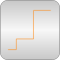# Package Contents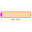MultiSwitch Set Integer expression that is associated with the first active input signal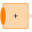Sum Sum of Integers: y = k*u + k*u + ... + k[n]*u[n]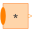Product Product of Integer: y = u*u* ... *u[n]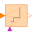TriggeredAdd Add input to previous value of output, if rising edge of trigger port

# Information

This information is part of the Modelica Standard Library maintained by the Modelica Association.

This package contains basic mathematical operations on Integer signals.